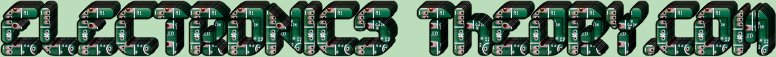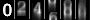# Series and Parallel Resistances - a Summary

To summarize all that we have just learned:

• There are 2 types of circuits.... Series and Parallel.
• Series Circuits
• Are connected in a straight line, like a chain.
• All current remains the same throughout the circuit.
• I Total = I 1 =I 2 =I 3 etc...
• There can be many different voltages in a series circuit, as a voltage drop appears across every resistor.
• The total voltage in a series circuit is equal to the sum of all the individual voltage drops within the circuit.
• E Total = E 1 + E 2 + E 3 + etc...

• The total resistance in a series circuit is equal to the sum of all the individual resistances within the circuit.
• The formula for Resistance in Series is:
R Total = R 1 + R 2 + R 3 + etc...

• Parallel Circuits
• Are connected allowing multiple paths for current flow.
• All voltage remains the same throughout the circuit.
• E Total = E 1 =E 2 =E 3 etc...
• There can be many different currents in a parallel circuit, as each leg has the same voltage, but can have a different resistance.
• The total current in a parallel circuit is equal to the sum of all the individual currents on each leg of the circuit.
• The formula for Current in Parallel is:
I RTotal = I R1 + I R2 + I R3 + etc...

• Resistance is found by reciprocating the sum of the reciprocals of the resistance of the individual branches
• The formula for Resistance in Parallel is:
1
-----------------------------------
1       1       1       1       1
---- + ---- + ---- + ---- + ---- +
R 1     R 2    R 3    R 4    R X...
• Ohm's Law states that there is a relationship which exists between current, resistance, and voltage, such that E = I x R
 (On The Following Indicator... PURPLE will indicate your current location) 1 2 3 4 5 6 7 8 9 10 11 12 13 14 15 16 17 18 19 20 21 22 23 24 25 26 27 28 29 30 31 32 33 34 35 36 37 38 39 40 41 42 43 44 45 46 47 48 49 50 51 52 53 54 55 56 57 58 59 60 61 62 63 64 65 66 67 68 69 70 71 72 73 74 75

[COURSE INDEX] [ELECTRONICS GLOSSARY] [HOME]Otherwise - please click to visit an advertiser so they know you saw their ad!

VISITORS: